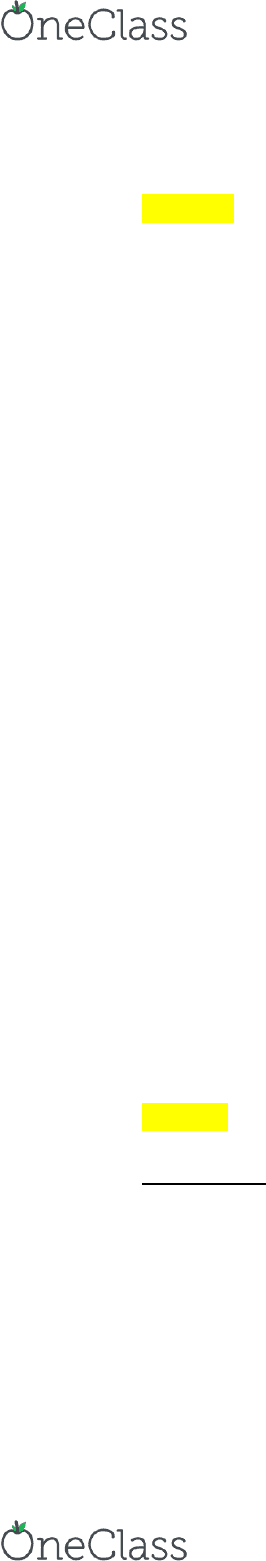# FIN 3410 Lecture 3: Day 3 Notes

9 views3 pages
School
Department
Course

For unlimited access to Class Notes, a Class+ subscription is required.Day 3 Notes
EX 6.1-3: Covered Interest Arbitrage
1. Borrow \$1,500,000 for 3m @ i\$3m = 2%
a. \$(loan + interest) = \$1,500,000 * (1 + .02)
b. = \$1,530,000
2. Exchange borrowed \$1,500,000 for L @ s = \$1.50/L
a. \$1,500,000 / (\$1.50/L) = 1,000,000 L
3. Invest 1,000,000 L in the UK
a. FV(UK1L) = 1,000,000 L * (1 + 0.0145)
b. = 1,014,500 L
4. To protect against possible L depreciation, sell FV(UK1L) forward for \$ @ F =
\$1.52/L
a. 1,014,500 L * (\$1.52/L) = \$1,542,040
5. Pay back \$(loan + interest) @ \$1,530,000
a. Arbitrage profit = \$ proceeds - \$ costs
b. \$1,542,040 - \$1,530,000 = \$12,040
i\$3m = 2% > iL3m = 1.45%
o Invest in the UK since L is expected to appreciate
How will IRP be restored?
o (1 + i\$3m) / (1 + iL3m) < F3m(\$/L) / S(\$/L)
1.0054 < 1.0133
o IRP will be restored if inequality becomes equality
(1 + i\$3m) / (1 + iL3m) GO UP
F3m(\$/L) / S(\$/L) GO DOWN
PPP and Exchange Rate Determination
I\$ = 3% and IE = 5%
So = \$1.25/E
\$1.25/E * (1.03) * (1.05)
PPP
o (1 + I\$) / (1 + IE) = F1(\$/E) / So(\$/E)
o E[St(\$/E)] = [(1 + I\$) / (1 + IE)]^t * So(\$/E)
EX 6.1-1
Background
V\$ = \$1,000,000 extra cash reserve
T = 6 months
i\$ = 8% per annum
o I\$6m = 8%/2 = 4%
iE = 7% per annum
o I\$6m = 7%/2 = 3.5%
So = E1.01/\$
F6m = E0.99/\$
find more resources at oneclass.com
find more resources at oneclass.com
Unlock document

This preview shows page 1 of the document.
Unlock all 3 pages and 3 million more documents.

Already have an account? Log in

# Get access

Grade+
\$10 USD/m
Billed \$120 USD annually
Homework Help
Class Notes
Textbook Notes
40 Verified Answers
Study Guides
1 Booster Class
Class+
\$8 USD/m
Billed \$96 USD annually
Homework Help
Class Notes
Textbook Notes
30 Verified Answers
Study Guides
1 Booster Class